## SOCNET@LISTS.UFL.EDU

#### View:

 Message:[First|Previous|Next|Last] By Topic:[First|Previous|Next|Last] By Author:[First|Previous|Next|Last] Font: Proportional Font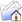LISTSERV Archives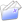SOCNET HomeSOCNET October 2005

Subject:Re: Brandes algorithm on betweeness centrality

From:Date:Wed, 5 Oct 2005 08:49:21 +0200

Content-Type:text/plain

Parts/Attachments: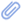text/plain (165 lines)
 ```***** To join INSNA, visit http://www.insna.org ***** On Tue, Oct 04, 2005 at 04:40:25PM -0700, phil wrote: > ***** To join INSNA, visit http://www.insna.org ***** > I googled and found this paper by Ulrik Brandes on a faster method to calculate betweeness centrality. http://www.inf.uni-konstanz.de/algo/publications/b-fabc-01.pdf I'm programming in Mathematica, and I entered and re-entered the algorithm multiple times, but the values produced are (very) slightly off from the betweeness values produced by the algorithm using conventional matrix multiplication and pair summations. Has anyone played aroudn with this thing and got it work? In addition to Vlado's comments, here are answers to the three most frequently asked questions about the algorithm. Best, B. Q1. Why do I get twice the values that UCINET gives me?   The way the algorithm is formulated, it considers ordered pairs of   vertices (which is necessary for directed graphs), so that each is   pair contributes twice to the betweenness of all other vertices.   If you have an undirected graph and do not normalize, simply divide   by two. Q2. Can I use it to compute edge betweenness?   Simply execute the following statement inside of the last for-loop:   C_B[v,w] = C_B[v,w] + sigma[v]/sigma[w] * (1+delta[w]) Q3. Do you have a reference implementation?   Here are C++ excerpts for betweenness in unweighted (BFS)   and weighted (Dijkstra) directed graphs (for undirected graphs   divide by 2, see above) using the LEDA library.   By the way: in weigthed graphs, the edge weight corresponds to a   *length*; if you want weights to reflect the *strength* of a tie,   use the version for unweighted graphs and multiply the shortest-path   count sigma with the strength of edges (i.e. interpret as multiplicity). //==================================================================== #include #include #include #include #include typedef list nodelist; // enables node_array> void betweenness(const graph& G, node_array& c) {   c.init(G, 0.0);   b_queue Q(G.number_of_nodes()); // BFS queue   b_stack S(G.number_of_nodes()); // order of discovery   node s; // source   forall_nodes(s, G)   {     Q.append(s);     node_array dist(G, -1); // distance from source     node_array sigma(G, 0); // number of shortest paths from source     node_array pred(G); // predecessors on any shortest paths     node_array delta(G, 0.0); // dependencies of source     dist[s] = 0; sigma[s] = 1;     while(!Q.empty()) // find and count shortest paths (BFS)     {       node v = Q.pop();       S.push(v); // remember for reverse traversal       edge e;       forall_out_edges(e,v)       {         node w = G.opposite(v,e);         if (dist[w] < 0) // new discovery?         {           Q.append(w);           dist[w] = dist[v]+1;         }         if (dist[w] > dist[v]) // first or subsequent discovery?         {           sigma[w] += sigma[v];           pred[w].append(v);         }       }     }     while(!S.empty()) // accumulate dependencies     {       node w = S.pop();       while(!pred[w].empty())       {         node v = pred[w].pop();         delta[v] += sigma[v]*(1.0+delta[w]) / (double)sigma[w];       }       if(w != s) c[w] += delta[w]; // source does not depend on itself     }   } } void weighted_betweenness(const graph& G,                           const edge_array& w,                           node_array& c) {   c.init(G, 0.0);   node_pq PQ(G);   b_stack S(G.number_of_nodes());   node s;   forall_nodes(s, G)   {     node_array dist(G, -1); // distance from source     node_array sigma(G, 0); // number of shortest paths from source     node_array pred(G); // predecessors on any shortest paths     node_array delta(G, 0.0); // dependencies of source     dist[s] = 0; sigma[s] = 1;     PQ.insert(s, 0);     while(!PQ.empty())     {       node u = PQ.del_min();       S.push(u);       edge e;       long du = dist[u];       forall_adj_edges(e, u)       {         node v = G.opposite(u,e);         long du_we = du + w[e];         if (sigma[v] == 0) // first discovery?           PQ.insert(v, du_we);         else           if (du_we < dist[v]) // shorter path discovered?           {             PQ.decrease_p(v, du_we);             pred[v].clear();             sigma[v] = 0;           } else             if (du_we > dist[v])               continue; // do not increase number of shortest paths         dist[v] = du_we;         sigma[v] += sigma[u];         pred[v].append(u);       }     }     while(!S.empty()) // accumulate dependencies     {       node v = S.pop();       while(!pred[v].empty())       {         node u = pred[v].pop();         delta[u] += sigma[u]*(1.0+delta[v]) / (double)sigma[v];       }       if(v != s) c[v] += delta[v]; // source does not depend on itself     }   } } //==================================================================== _____________________________________________________________________ SOCNET is a service of INSNA, the professional association for social network researchers (http://www.insna.org). To unsubscribe, send an email message to [log in to unmask] containing the line UNSUBSCRIBE SOCNET in the body of the message.```

#### Search Archives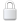Log In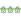Get Password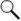Search Archives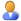Subscribe or Unsubscribe